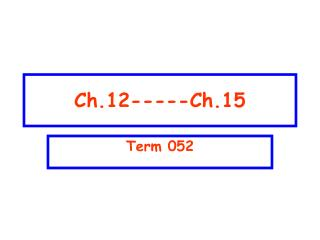DownloadDownload PresentationCh.12-----Ch.15

# Ch.12-----Ch.15

Télécharger la présentation## Ch.12-----Ch.15

- - - - - - - - - - - - - - - - - - - - - - - - - - - E N D - - - - - - - - - - - - - - - - - - - - - - - - - - -
##### Presentation Transcript

1. Ch.12-----Ch.15 Term 052

2. T052: Q#16:A 5.0-m weightless rod (AC), hinged to a wall at A, is used to support an 800-N block as shown in Fig 5. The horizontal and vertical components of the force (FH, FV) of the hinge on the rod are: (Ans: FH = 600 N, FV = 800N ) Q#17:A shearing force F = 50 N is applied to an aluminum rod with a length of L =10 m, a cross-sectional area A=1.0 × 10–5 m2, and a shear modulus G = 2.5 × 1010 N/m2. As a result the rod is sheared through a distance (Δx) of: (Ans: 0.20 cm) Q#18: A man holds a rod AB of length = 6.0 m and weight = 30 N in equilibrium, by exerting an upward force F1, with one hand, and a downward force F2, with the other hand as shown in Fig 6. What are the magnitude of the forces F1 and F2? (Ans: F1 = 90 N, F2 = 60 N) Ch-12

3. T052 Q#19: Three identical particles each of mass m are distributed along the circumference of a circle of radius R as shown in Fig 7. The gravitational force of m2 on m1 is 1.0 x 10-6 N. The magnitude of the net gravitational force on m1 due to m2, m3 is (Ans: 1.4 x 10-6 N) Q#20: Calculate the mass of the Sun using the fact that Earth is rotating around the Sun in a circular orbit of radius 1.496 x 1011m with a period of one year (1 year =3.156 x 107s).(Ans: 1.99 x 1030 kg). Q#21: Calculate the work require to move an Earth satellite of mass m from a circular orbit of radius 2RE to one of radius 3RE. (Consider ME = mass of Earth, RE = radius of Earth, G = universal Gravitational constant) (Ans: G ME m /(12 RE)). Q#22: A 100-kg rock from outer space is heading directly toward Earth. When the rock is at a distance of (9RE) from the Earth's surface, its speed is 12 km/s. Neglecting the effects of the Earth's atmosphere on the rock, find the speed of the rock just before it hits the surface of Earth.(Ans: 16 km/s) Ch-13

4. T052 Q#23: A uniform U-tube is partially filled with water. Oil, of density 0.75 g/cm3, is poured into the right arm until the water level in the left arm rises 3.0 cm (see Fig 8). The length of the oil column (h) is then: (Ans: 4.0 cm) Q#24: The dimensions of a boat (ρboat= 150 kg/m3) is 3.00 m x 3.00 m x 1.00 m. What maximum load can it carry in sea water (ρsea water= 1020 kg/m3) without sinking? (Ans: 7830 kg) Q#25: A water line enters a house 2.0 m below ground. A smaller diameter pipe carries water to a faucet 5.0 m above ground, on the second floor. Water flows at 2.0 m/s in the main line and at 7.0 m/s on the second floor. If the pressure in the main line is 3.0 x 105 Pa, then the pressure on the second floor is: (Take the density of water to be 1.0 x 103 kg/m3) (Ans: 2.1 x 105 P) Q#26: The rate of flow of water through a horizontal pipe is 2.00 m3/min. Calculate the speed of flow at a point where the diameter of the pipe is 10.0 cm. (Ans: 4.24 m/s). Ch-14

5. T052 Q#27: A mass m1 = 1.0 kg is connected to a spring (with spring constant equal to k) and oscillates on a horizontal frictionless table with a period of 1.0 s. When m1 is replaced with another unknown mass m2, the period changes to 2.0 s. Find the value of m2.n (Ans: 4.0 kg) Q#28: A 0.500 kg block is connected to a spring (k = 20.0 N/m) and oscillates on a horizontal frictionless table. Calculate the maximum kinetic energy of the block if the amplitude of the simple harmonic motion is 3.00 cm. (Ans: 9.00 x 10-3 J) Q#29: If the displacement of a block-spring system is described by the following equation: x(t)=0.2cos(10t)where x is in m, and t is in s. What is the speed of the block when its displacement is x = 0.1 m? (Ans: 1.73 m/s) Q#30: A simple pendulum has a period of 10.0 s if the free fall acceleration is g. What would its period be if the free fall acceleration is g/2? (Ans: 14.1 s) Ch-15